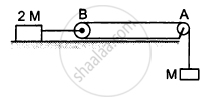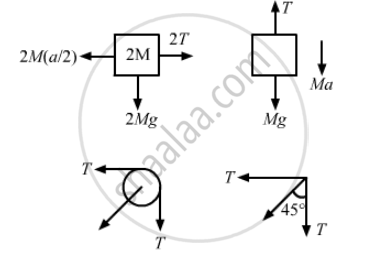# Consider the Situation Shown in the Following Figure. Both the Pulleys and the String Are Light and All the Surfaces Are Frictionless. (A) Find the Acceleration - Physics

Sum

Consider the situation shown in the following figure. Both the pulleys and the string are light and all the surfaces are frictionless. (a) Find the acceleration of the mass M; (b) find the tension in the string; (c) calculate the force exerted by the clamp on the pulley A in the figure.#### Solution

Let the acceleration of mass M be a.
So, the acceleration of mass 2M will be $\frac{a}{2}$(a) 2M(a/2) − 2T = 0
⇒ Ma = 2T
T + Ma − Mg = 0
$\Rightarrow \frac{Ma}{2} + Ma = Mg$
$\Rightarrow 3Ma = 2Mg$
$\Rightarrow a = \frac{2g}{3}$

(b) Tension,
$T = \frac{Ma}{2} = \frac{M}{2} \times \frac{2g}{3} = \frac{Mg}{3}$

(c) Let T' = resultant of tensions

$\therefore T' = \sqrt{T^2 + T^2} = \sqrt{2}T$

$\therefore T' = \sqrt{2}T = \frac{\sqrt{2}Mg}{3}$

$\text{Again, }\tan\theta = \frac{T}{T} = 1$

$\Rightarrow \theta = 45^\circ$

So, the force exerted by the clamp on the pulley is (sqrt2"Mg")/3 at an angle of 45° with the horizontal.

Concept: Newton’s Second Law of Motion
Is there an error in this question or solution?

#### APPEARS IN

HC Verma Class 11, Class 12 Concepts of Physics Vol. 1
Chapter 5 Newton's Laws of Motion
Q 31 | Page 81